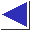# comp.lang.c FAQ list ·Question 20.3

Q: How can I open files mentioned on the command line, and parse option flags?

A: Here is a skeleton which implements a traditional Unix-style argv parse, handling option flags beginning with -, and optional filenames. (The two flags accepted by this example are -a and -b; -b takes an argument.)

```#include <stdio.h>
#include <string.h>
#include <errno.h>

main(int argc, char *argv[])
{
int argi;
int aflag = 0;
char *bval = NULL;

for(argi = 1; argi < argc && argv[argi] == '-'; argi++) {
char *p;
for(p = &argv[argi]; *p != '\0'; p++) {
switch(*p) {
case 'a':
aflag = 1;
printf("-a seen\n");
break;

case 'b':
bval = argv[++argi];
printf("-b seen (\"%s\")\n", bval);
break;

default:
fprintf(stderr,
"unknown option -%c\n", *p);
}
}
}

if(argi >= argc) {
/* no filename arguments; process stdin */
printf("processing standard input\n");
} else {
/* process filename arguments */

for(; argi < argc; argi++) {
FILE *ifp = fopen(argv[argi], "r");
if(ifp == NULL) {
fprintf(stderr, "can't open %s: %s\n",
argv[argi], strerror(errno));
continue;
}

printf("processing %s\n", argv[argi]);

fclose(ifp);
}
}

return 0;
}
```
(This code assumes that fopen sets errno when it fails, which is not guaranteed, but usually works, and makes error messages much more useful. See also question 20.4.)

There are several canned functions available for doing command line parsing in a standard way; the most popular one is getopt (see also question 18.16). Here is the above example, rewritten to use getopt:

```extern char *optarg;
extern int optind;

main(int argc, char *argv[])
{
int aflag = 0;
char *bval = NULL;
int c;

while((c = getopt(argc, argv, "ab:")) != -1)
switch(c) {
case 'a':
aflag = 1;
printf("-a seen\n");
break;

case 'b':
bval = optarg;
printf("-b seen (\"%s\")\n", bval);
break;
}

if(optind >= argc) {
/* no filename arguments; process stdin */
printf("processing standard input\n");
} else {
/* process filename arguments */

for(; optind < argc; optind++) {
FILE *ifp = fopen(argv[optind], "r");
if(ifp == NULL) {
fprintf(stderr, "can't open %s: %s\n",
argv[optind], strerror(errno));
continue;
}

printf("processing %s\n", argv[optind]);

fclose(ifp);
}
}

return 0;
}
```

The examples above overlook a number of nuances: a lone ``-'' is often taken to mean ``read standard input''; the marker ``--'' often signifies the end of the options (proper versions of getopt do handle this); it's traditional to print a usage message when a command is invoked with improper or missing arguments.

If you're wondering how argv is laid out in memory, it's actually a ``ragged array''; see the picture in question 20.2. See also questions 8.2, 13.7, and 19.20.

References: K&R1 Sec. 5.11 pp. 110-114
K&R2 Sec. 5.10 pp. 114-118
ISO Sec. 5.1.2.2.1
H&S Sec. 20.1 p. 416
PCS Sec. 5.6 pp. 81-2, Sec. 11 p. 159, pp. 339-40 Appendix F
Schumacher, ed., Software Solutions in C Sec. 4 pp. 75-85